# About

The paper “QuNet: Cost vector analysis & multi-path entanglement routing in quantum networks” (https://arxiv.org/abs/2105.00418) presents the theory, design and initial results for QuNet.

Developers:

# Goal

QuNet is a highly-scalable, multi-user quantum network simulator and benchmarking tool implemented in Julia. It relies on efficient algorithms for performing multi-user routing and congestion avoidance using quantum memories. QuNet focusses on the specific task of distributing entangled Bell pairs between users ($$A$$ and $$B$$),

$$|\Psi^+\rangle = \frac{1}{\sqrt{2}}(|0\rangle_A|0\rangle_B + |1\rangle_A|1\rangle_B).$$

These can subsequently be employed in state teleportation protocols to transmit arbitrary quantum states, or be applied to quantum key distribution, distributed quantum computing, or other entanglement-based quantum protocols.

QuNet uses a cost vector methodology. Rather than track quantum states themselves, we track their associated costs as they traverse the network. Costs are arbitrary properties that accumulate additively as qubits traverse networks. We can express physical degradation such as loss, dephasing or depolarising processes in this form, and also non-physical costs such as monetary ones. Tracking the accumulation of costs acting on Bell pairs is equivalent to directly tracking the states themselves.

# Quantum channels

Quantum channels can be represented in the quantum process formalism using the Kraus (or operator-sum) representation. In many useful instances these can be converted to additive metrics amenable to our cost vector formalism.

Consider the loss channel,

$$\mathcal{E}_\mathrm{loss}(\rho) = p\rho + (1-p)|vac\rangle\langle vac|,$$

where $$p$$ is the probability of a qubit not being lost, otherwise replaced with the vacuum state $$|vac\rangle$$. If multiple such channels are applied in series the $$p$$’s multiply,

$$p_\mathrm{total} = \prod_i p_i.$$

However by converting to logarithmic form we can make this additive,

$$-\log(p_\mathrm{net}) = -\sum_i \log(p_i).$$

Now $$m_i=-\log(p_i)$$ can be used as an additive edge weight. This is a common approach amongst experimentalists, who typically consider loss over fibre in terms of decibels per unit distance (dB/m).

We can apply a similar approach to depolarising channels whose quantum process is given by,

$$\mathcal{E}_\mathrm{depol}(\rho) = p\rho + (1-p)\frac{I}{2},$$

and $$m=-\log(p)$$ acts additively. With some algebraic manipulation we can apply this to dephasing channels,

$$\mathcal{E}_\mathrm{deph}(\rho) = (2p-1)\rho + (1-p)(\rho + Z\rho Z),$$

where $$-\log(2p-1)$$ acts as our additive dephasing metric. Note this representation of the dephasing channel has been algebraically manipulated into an unaffected term ($$\rho$$), and a completely dephased term ($$\rho+Z\rho Z$$) which is the steady-state of the channel. This approach could also be applied to bit-flip ($$X$$) channels, bit-phase-flip ($$Y$$) channels, or amplitude damping channels.

# Multi-path routing

Classical networks rely on path-finding algorithms (e.g shortest path à la Dijkstra) for optimal packet routing. This is highly efficient, since Dijkstra’s algorithm has worst case $$O(V^2)$$ runtime in the number of graph vertices $$V$$. Quantum networks can employ multi-path routing, whereby multiple independently routed Bell pairs are purified into one of higher fidelity.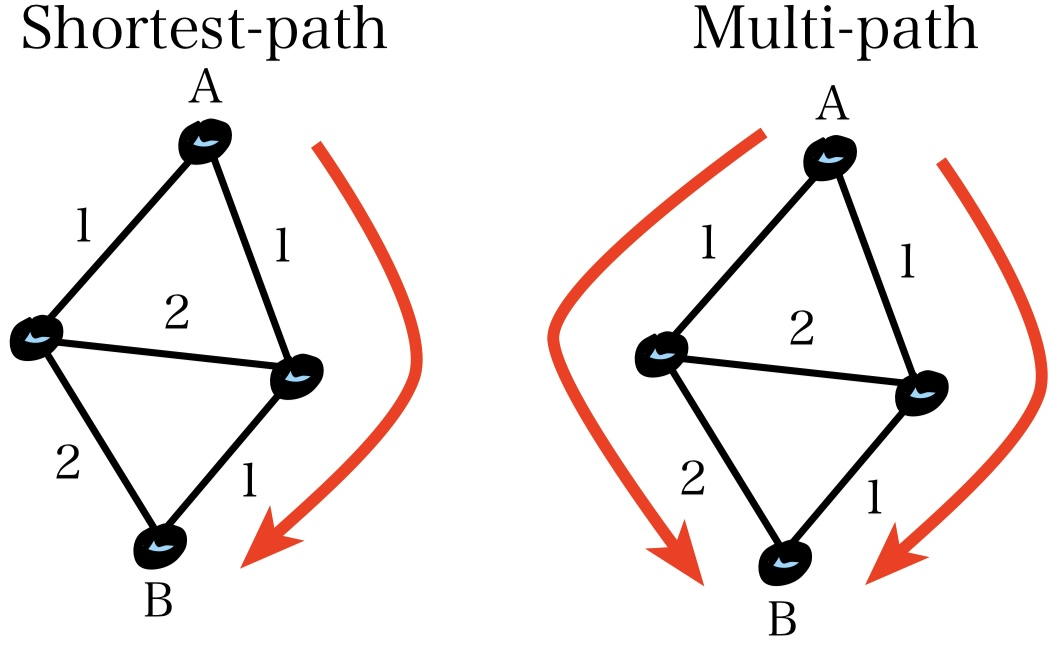We implement multi-path routing via multiple application of shortest path routing, where subsequent rounds exclude previously consumed routes from consideration.

# Entanglement swapping & purification

Primitive operations in quantum networks include entanglement swapping (for extending entanglement links), and entanglement purification (for boosting fidelity).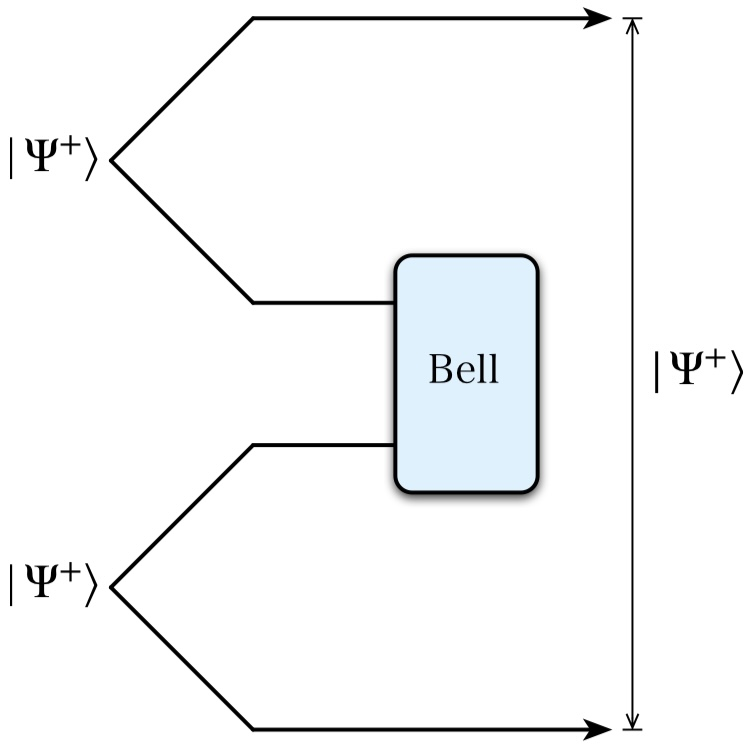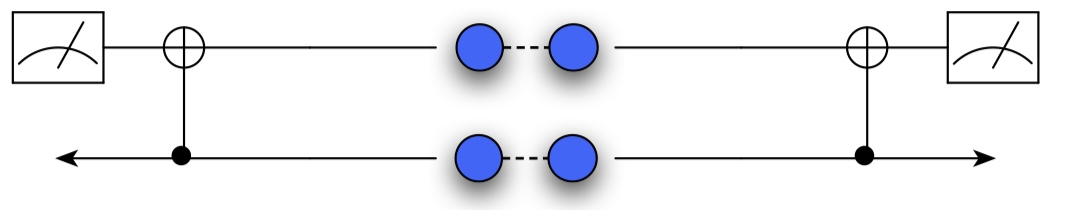# Graph reduction

These primitives provide simple substitution rules for graph reduction.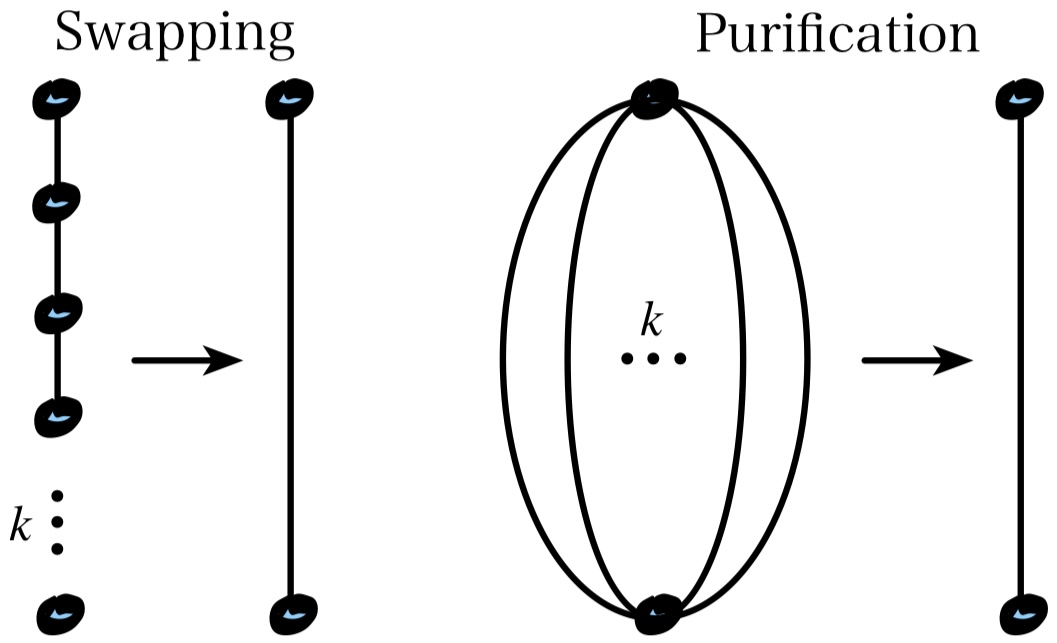# Network abstraction

Quantum repeaters, comprising classically-controlled (and sometimes randomised) sequences of swapping and purification operations, can be reduced to a single virtual link capturing their average cost vector, thereby bypassing the need for directly accommodating non-deterministic or classically-controlled operations, maintaining compatability with the QuNet framework. Similarly, SneakerNet channels, whereby a large number of error-corrected qubits are physically transported can be reduced to an equivalent cost vector too.

For large networks it may not always be necessary to understand the full dynamics across all nodes and channels. Instead we might focus higher-level abstractions of the network, which consider the dynamics between designated subnetworks or regions, reducing computational load.

# Space-based networks

QuNet can accommodate both static and dynamic nodes and channels. Here Alice & Bob have the option of communicating via:

• A static ground-based fibre link.
• A LEO satellite passing overhead through atmospheric free-space channels, which dynamically update.
• Exploiting both and purifying them together (multi-path routing).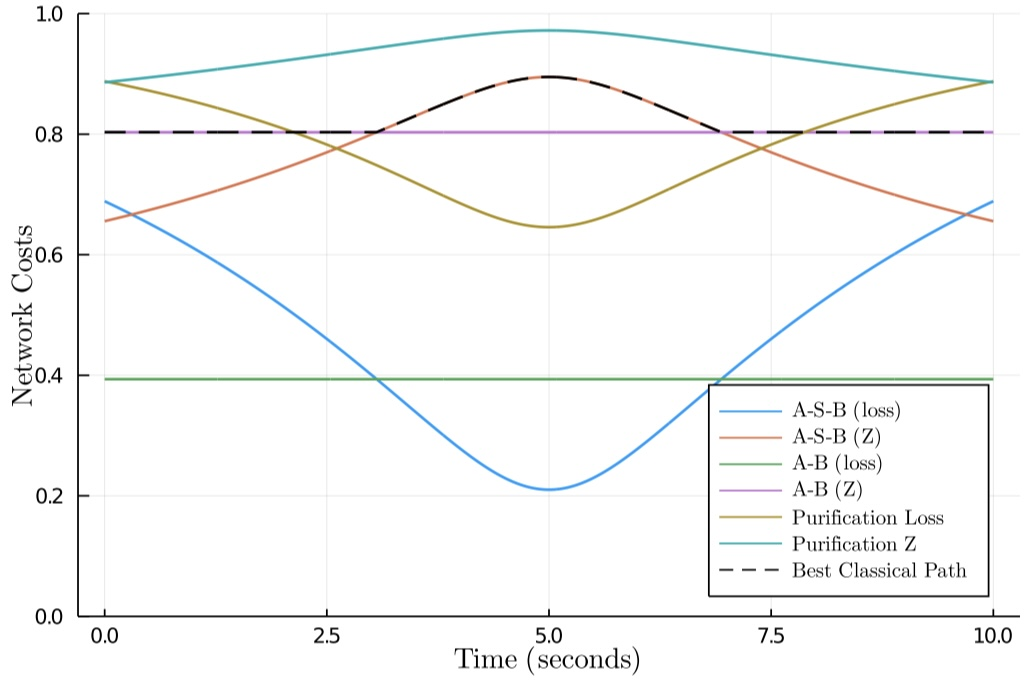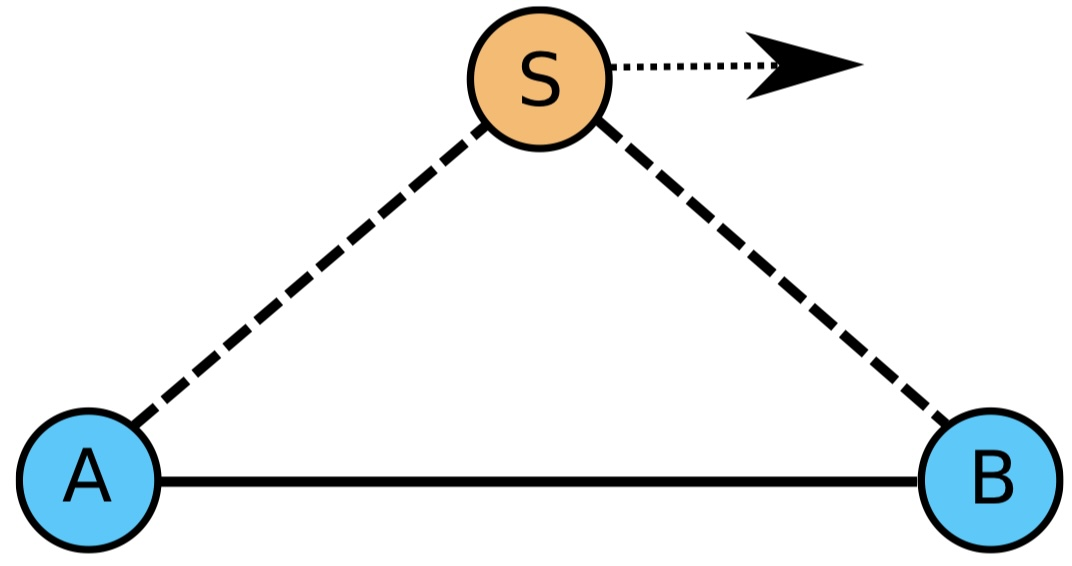# Code example

This is the QuNet code in Julia that creates that network. Julia modules can be called from Python or run in Jupyter notebooks too. You can learn more about the Julia language at www.julialang.org.

using QuNet

Q = QNetwork()
A = BasicNode("A")
B = BasicNode("B")
S = PlanSatNode("S")

B.location = Coords(500,0,0)
S.location = Coords(-2000,0,1000)
S.velocity = Velocity(1000,0)

AB = BasicChannel(A, B, exp_cost=true)
AS = AirChannel(A, S)
SB = AirChannel(S, B)

for i in [A, S, AB, AS, SB]
add(Q, i)
end


# Temporal routing & quantum memories

We accommodate for quantum memories by treating them as temporal channels between the respective nodes of identical copies of the underlying graph, where each layer represents the network at a particular point in time. This is far more efficient than naïve combinatoric congestion mitigation techniques, relying on the same underlying routing algorithms applied to a graph with a simple linear overhead in size.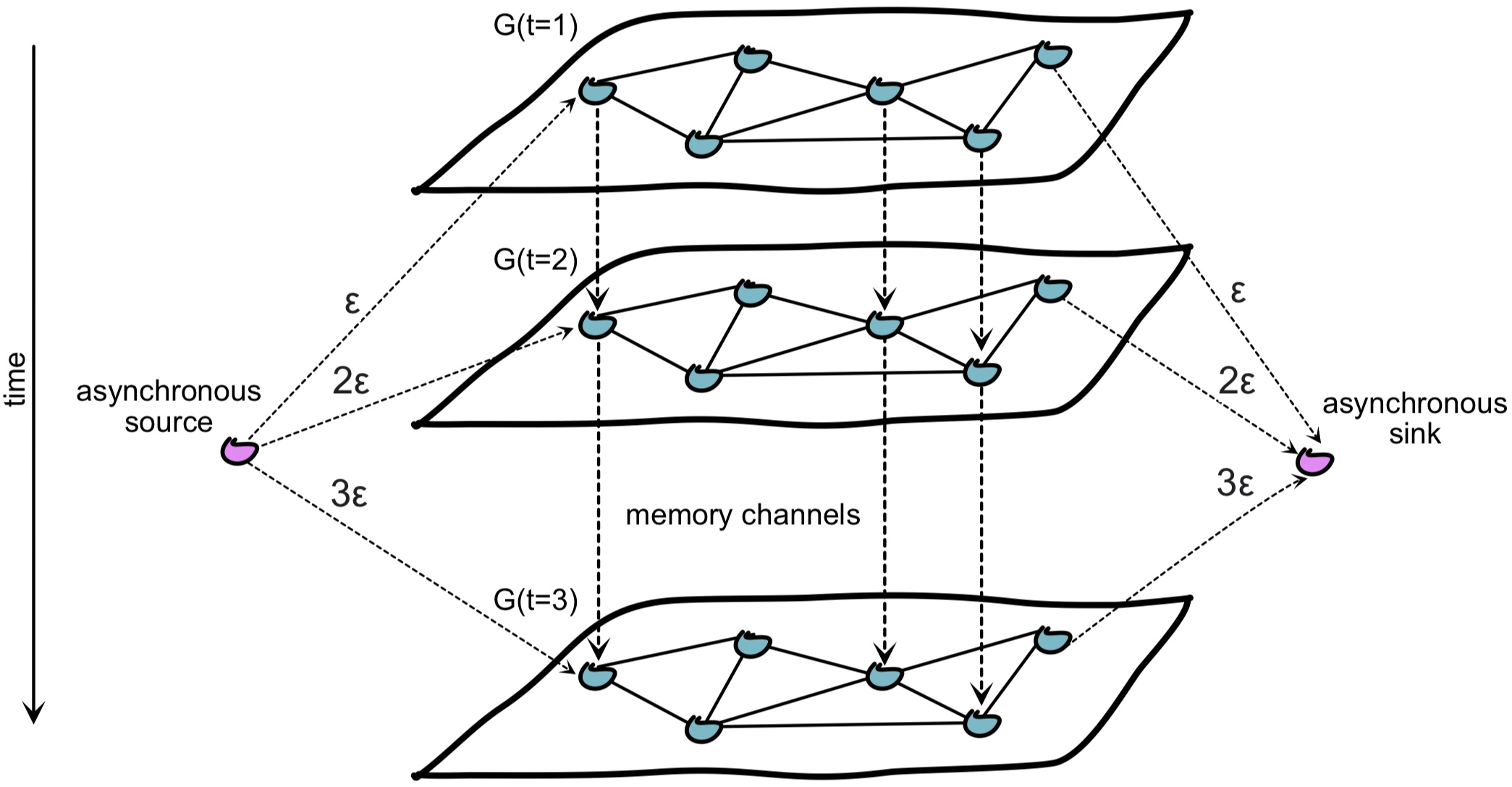The incrementally weighted asynchronous nodes guide the routing algorithm to preference earlier times, thereby temporally compressing multi-user routing, and providing a temporal routing queue.

The compression ratio is the ratio between routing time with and without memories. Here we show the temporal compression ratio of our algorithm against increasing network congestion.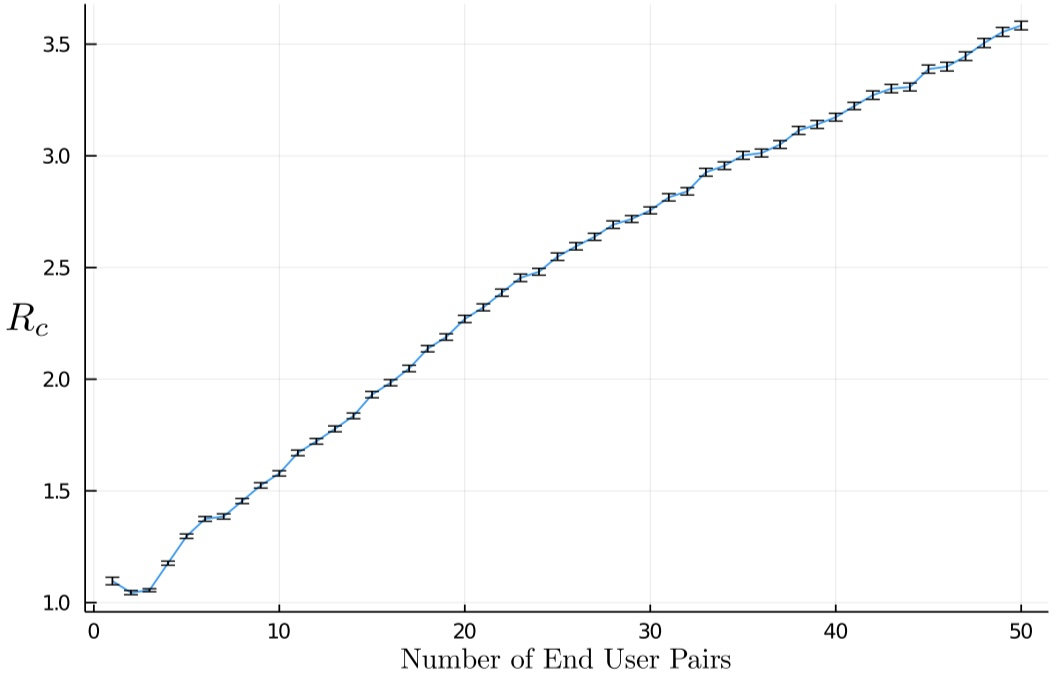Here’s a multi-user network with 3 users (colour coded) and multi-path routing (maximum 3 paths per user). The stacked layers represent time.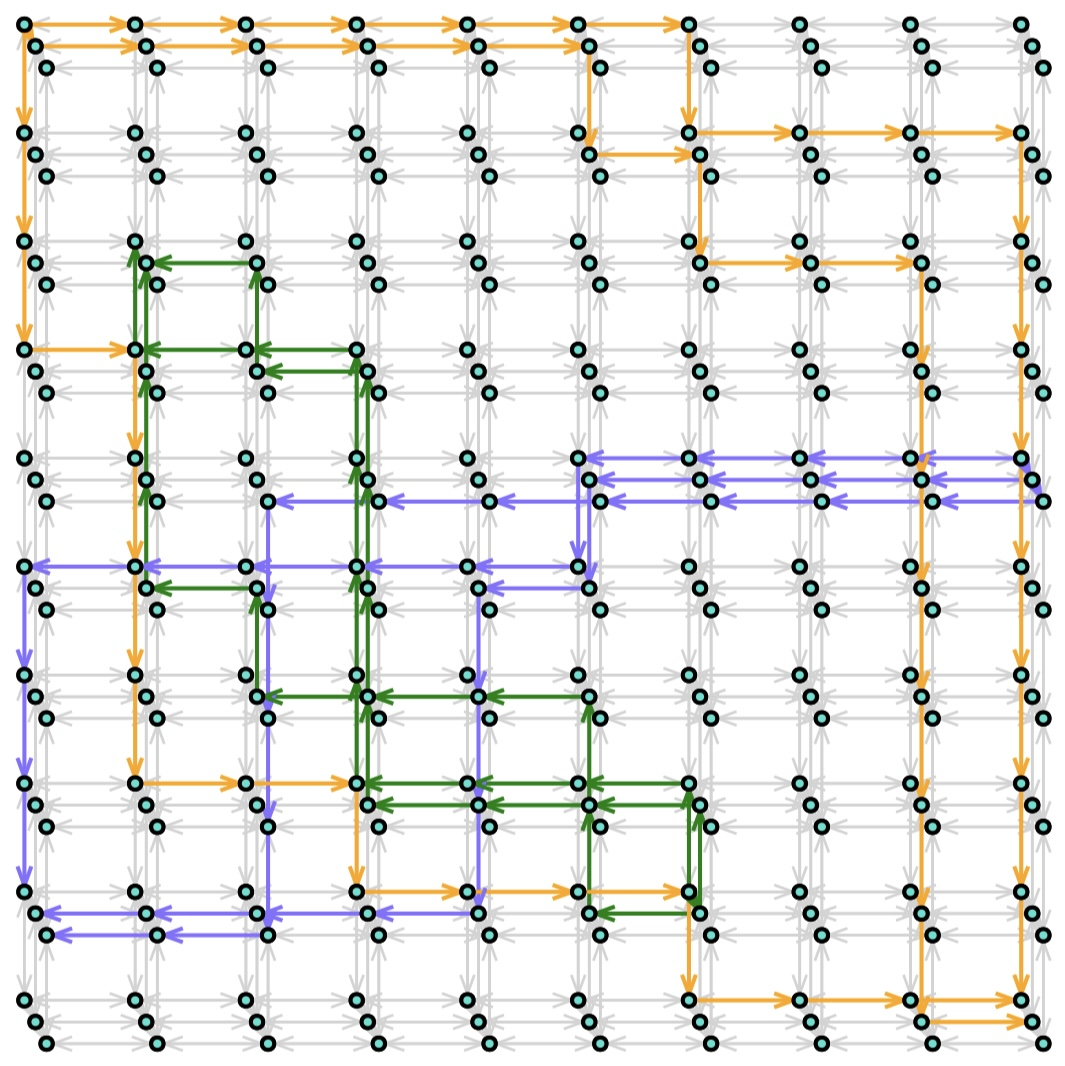# Efficient multi-path routing

Our greedy multi-path routing algorithm allows multi-user routing with congestion mitigation via quantum memories, with algorithmic efficiency,

$$O(M^3V^2),$$

for $$M$$ user-pairs on a $$V$$-vertex graph, and is therefore highly scalable and efficient in both users and network size.

Here we consider a grid network with edge percolations, showing the likelihood of users utilising different path numbers as the network becomes increasingly disconnected.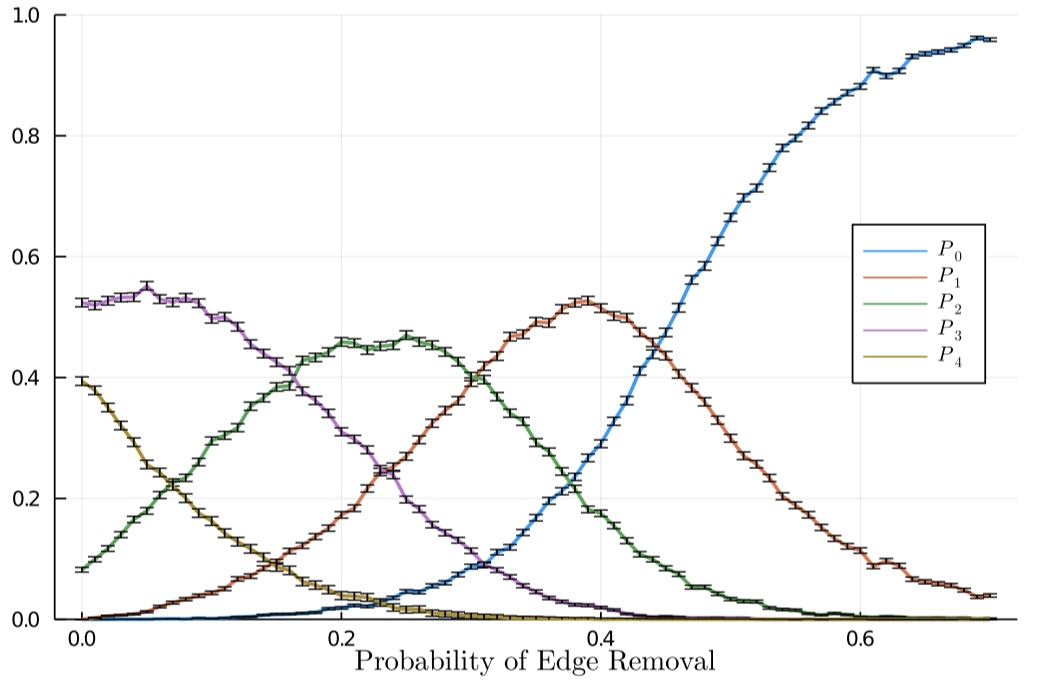# Application to quantum key distribution

This heat map shows the fidelity/efficiency trade off for random user pairs on a square lattice network. The distinct heat curves correspond to different numbers of paths utilised. Superimposed contours show achievable per-user E91 QKD secret key rates for the network.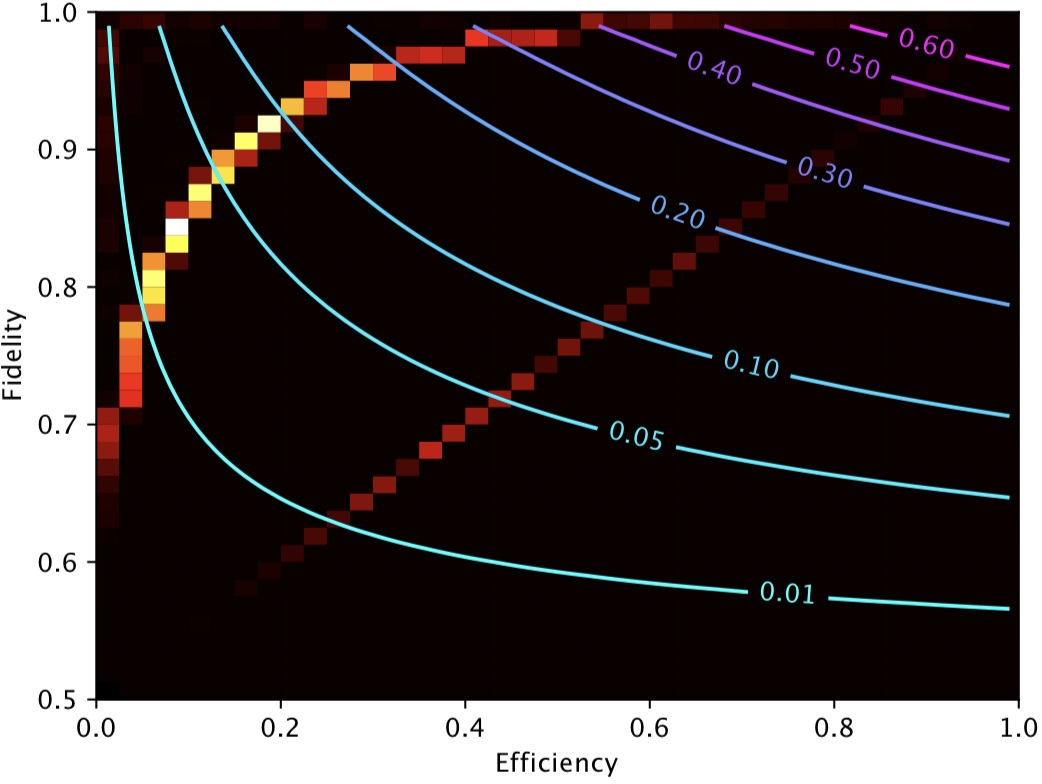# Application to distributed quantum computing

Our next stage of research is applying QuNet to distributed quantum computing. Entanglement links can be used to fuse together geographically separated graph states, facilitating distributed quantum computation exponentially more powerful than the sum of the parts.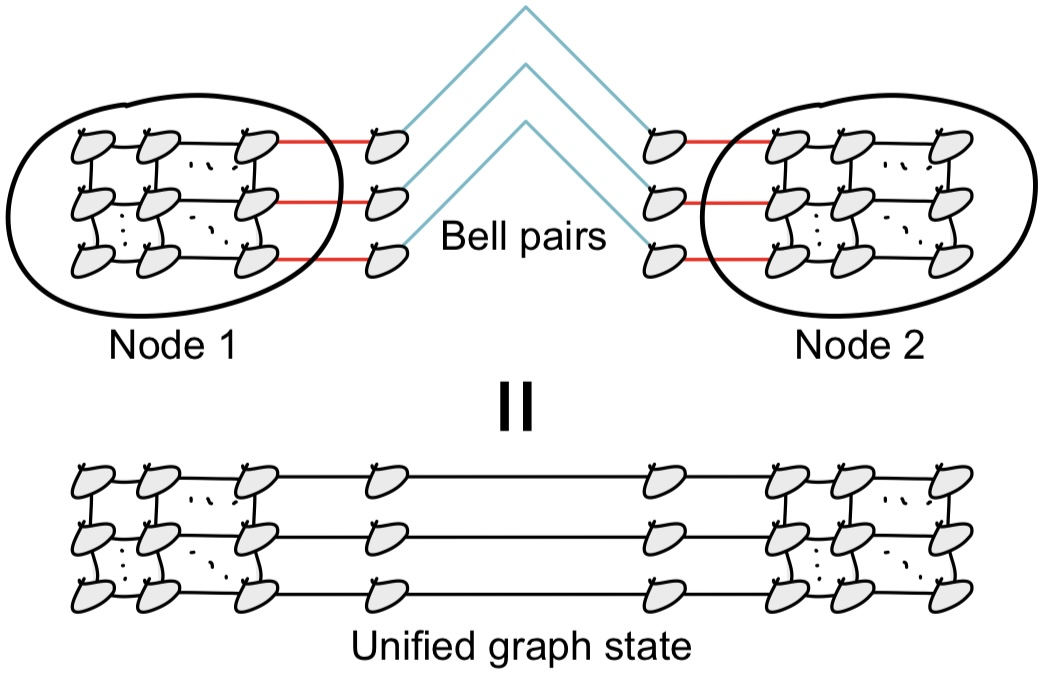Consider a distributed computer with N nodes, each with n bits/qubits, and a scaling function that indicates classical-equivalent compute power (classically this is linear, for quantum computers super-linear). The computational gain achieved by unifying remote devices is,

$$\lambda = \frac{f_\mathrm{sc}(Nn)}{N\cdot f_\mathrm{sc}(n)}.$$

Through unification of remote computational assets:

• Classical computers, $$\lambda=1$$. There is no computational enhancement.
• Quantum computers $$\lambda>1$$, in the best case $$\lambda=\mathrm{exp}(N)$$. We achieve exponential computational enhancement.

# The vision

In a future world where scalable quantum computers are available and ubiquitous it is clear that networking them together has the potential to provide exponentially more computational power than the sum of the parts. The big question is whether the cost of constructing the global quantum communications infrastructure necessary to facilitate this is justified by the economic return associated with this computational enhancement.

Our vision for the quantum internet is presented in the upcoming book “The Quantum Internet” published by Cambridge University Press.

# Acknowledgements

We thank Darcy Morgan, Alexis Shaw, Marika Kieferova, Zixin Huang, Louis Tessler, Yuval Sanders, Jasminder Sidhu, Simon Devitt & Jon Dowling for conversation (both helpful, unhelpful, meaningless, derogatory, and diatribe). We also thank the developers of JuliaGraphs, which QuNet makes heavy use of.